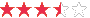Remember me

 Consumer Demand Forecasting: Popular Techniques, Part 2: Exponential Smoothing
Rating: 8 vote(s)Author: Eyal Eckhaus, posted on 6/25/2010 , in category "Logistics"
Abstract: Exponential smoothing techniques are simple, accurate and easy to understand demand forecasting techniques. This article is the second in a series of articles that demonstrate some popular forecasting techniques.

Exponential smoothing techniques are simple, accurate and easy to understand demand forecasting techniques. This article is the second in a series of articles that demonstrate some popular forecasting techniques.

1.  Exponential smoothing

Exponential smoothing and moving average are the two most common time-series forecasting methods , where exponential smoothing is one of the most widespread forecasting set of techniques, probably because of its simplicity, reasonable accuracy and computationally efficiency . Exponential smoothing is widely used as a forecasting technique in inventory systems and business planning , and its results are easy to understand and communicate .
This forecasting technique is the simplest of forecasting tools, combining recent forecasts with latest actual observations , but despite its simplicity it provides good practical results compared to more complicated smoothing and forecasting methods . Finally, it is easy to compute and proven to outperform other forecasting methods .

*Editor’s note: use the free online exponential smoothing generator to generate forecasts using the exponential smoothing technique.

Exponential smoothing uses three numbers :
- a smoothing constant (the weight assigned) – α,
- the previous forecast – Ft
- the previous period’s actual demand – At
The forecast equation of the simple exponential smoothing is:  α × demand in latest period + (1- α) × forecast for previous period. i.e.:
Ft+1 = α×At + (1- α) ×Ft

2.  Selecting of the value of α

The value of the smoothing parameter α ranges between zero and one . The higher it is, the more sensitive the forecast to change; however too high α value will produce unreliable results because it will depend too much on demand in the latest period. Therefore, an effective range of values for α may be 0.1-0.4, where the higher the demand variance, the higher α .  Selecting the optimal value for the number of past observations can be done with the MAD examination, explained in article .
Table 1: Example of exponential smoothing forecast with α=0.1
MonthDemand (thousands)Prediction with α = 0.1
1 21 21.00
2 18 21.00
3 35 20.70
4 26 22.13
5 33 22.52
6 23 23.57
7 43 23.51
8 22 25.46
9 33 25.11
10 23 25.90
11 42 25.61
12 ? 27.25

The 12-month demand prediction is a recursive result of calculating the prediction for all previous observations. The 12-month prediction calculation is: 0.1 × 42 + (1- 0.1) × 25.61 = 27.25

3.  Summary

The exponential smoothing technique is one of the most popular methods for predicting demand. This article demonstrated its calculation, and is the second in the article set describing forecasting techniques.

Part 1 introduces the demand forecasting issue, and demonstrated the weighted and unweighted moving average technique
Part 3 presents regression analysis
Part 4 discusses selecting among all techniques

4.  Bibliography

1. Nachtmann, H., M.A. Waller, and D.W. Rieske, The impact of point-of- sale data innacuracy and inventory record data errors. Journal of Business Logistics, 2010. 31(1): p. 149-IX.
2. Wikner, J., Analysis of smoothing techniques: application to production-inventory systems. Kybernetes, 2006. 35(9): p. 1323- 1347.
3. Bermúdez, J.D., J.V. Segura, and E. Vercher, Improving demand forecasting accuracy using nonlinear programming software. The Journal of the Operational Research Society, 2006. 57(1): p. 94.
4. Broze, L. and G. Melard, Exponential Smoothing: Estimation by Maximum Likelihood. Journal of Forecasting, 1990. 9(5): p. 445.
5. Wild, T., Best practices in inventory management 1997: Chapter 10. Wiley publication.
6. Cipra, T., Robust Exponential Smoothing. Journal of Forecasting, 1992. 11(1): p. 57.
7. Lawrence, F.B., Closing the logistics loop: A tutorial. Production and Inventory Management Journal, 1999. 40(1): p. 43-51.
8. Balaban, E., A. Bayar, and R.W. Faff., Forecasting stock market volatility: Further international evidence. The European Journal of Finance, 2006. 12(2): p. 171-188.
9. Eckhaus, E. (2010, June 25). Consumer Demand Forecasting: Popular Techniques, Part 4: Selecting the Optimal Method. Retrieved June 25, 2010 from http://www.purchasesmarter.com/articles/121

copyright © Purchasesmarter.com. The material may not be published, rewritten, broadcast, or redistributed. Any reproduction in whole or part by and individuals or organizations will be held liable for copyright infringement to the full extent of the law.

Rate

User Feedback